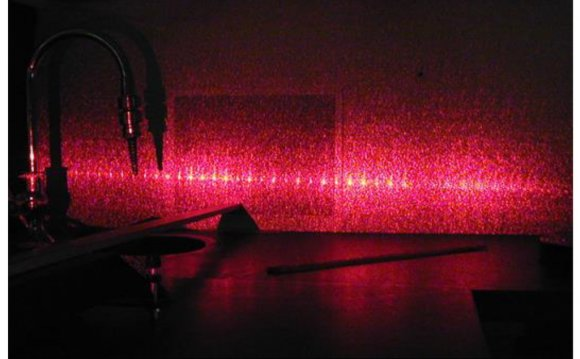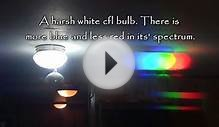# Diffraction grating element

September 22, 2022A very large reflecting diffraction grating.

In optics, a diffraction grating is an optical component with a regular pattern, which splits (diffracts) light into several beams travelling in different directions. The directions of these beams depend on the spacing of the grating and the wavelength of the light so that the grating acts as a dispersive element. Because of this, gratings are commonly used in monochromators and spectrometers.

A photographic slide with a fine pattern of black lines forms a simple grating. For practical applications, gratings generally have grooves or rulings on their surface rather than dark lines. Such gratings can be either transparent or reflective. Gratings which modulate the phase rather than the amplitude of the incident light are also produced, frequently using holography.

The principles of diffraction gratings were discovered by James Gregory, about a year after Newton's Prism experiments, initially with artifacts such as bird feathers. The first man-made diffraction grating was made around 1785 by Philadelphia inventor David Rittenhouse, who strung hairs between two finely threaded screws. This was similar to notable German physicist Joseph von Fraunhofer's wire diffraction grating in 1821.

### Theory of operation

The relationship between the grating spacing and the angles of the incident and diffracted beams of light is known as the grating equation.

When a wave propagates, each point on the wavefront can be considered to act as a point source, and the wavefront at any subsequent point can be found by adding together the contributions from each of these individual point sources.

An idealized grating is considered here which is made up of a set of long and infinitely narrow slits of spacing . When a plane wave of wavelength λ, is incident normally on the grating, each of the point slits in the grating acts as a set of point sources which propagate in all directions. The light in a particular direction, θ, is made up of the interfering components from each slit. Generally, the phases of the waves from different slits will vary from one another, and will cancel one another out partially or wholly. However, when the path difference between the light from adjacent slits is equal to the wavelength, λ, the waves will all be in phase. This occurs at angles m which satisfy the relationship sinm/λ=|| where is the separation of the slits and is an integer. Thus, the diffracted light will have maxima at angles m given by

d sin{theta_m} = m lambda.

It is straightforward to show that if a plane wave is incident at an angle θi, the grating equation becomes

d left( sin{theta_m} + sin{theta_i} right) = m lambda.

The light that corresponds to direct transmission (or specular reflection in the case of a reflection grating) is called the zero order, and is denoted = 0. The other maxima occur at angles which are represented by non-zero integers . Note that can be positive or negative, resulting in diffracted orders on both sides of the zero order beam.

This derivation of the grating equation has used an idealized grating. However, the relationship between the angles of the diffracted beams, the grating spacing and the wavelength of the light apply to any regular structure of the same spacing, because the phase relationship between light scattered from adjacent elements of the grating remains the same. The detailed distribution of the diffracted light depends on the detailed structure of the grating elements as well as on the number of elements in the grating, but it will always give maxima in the directions given by the grating equation.

Gratings can be made in which various properties of the incident light are modulated in a regular pattern; these include

• transparency (transmission amplitude gratings)
• reflectance (reflection amplitude gratings)
• direction of optical axis (optical axis gratings)

The grating equation applies in all these cases.

### Gratings as dispersive elements

The wavelength dependence in the grating equation shows that the grating separates an incident polychromatic beam into its constituent wavelength components, i.e., it is dispersive. Each wavelength of input beam spectrum is sent into a different direction, producing a rainbow of colors under white light illumination. This is visually similar to the operation of a prism, although the mechanism is very different.

A light bulb of a flashlight seen through a transmissive grating, showing three diffracted orders. The order = 0 corresponds to a direct transmission of light through the grating. In the first positive order ( = +1), colors with increasing wavelengths (from blue to red) are diffracted at increasing angles.

The diffracted beams corresponding to consecutive orders may overlap, depending on the spectral content of the incident beam and the grating density. The higher the spectral order, the greater the overlap into the next order.